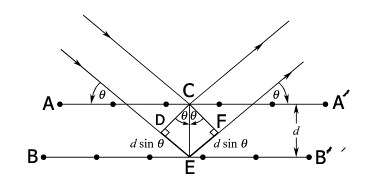1. Home
2. Yarn Engineering
3. Textile Physics-1
4. X-ray diffraction method# X-ray diffraction method

2.86K
0#### Md Sohanur Rahman Sobuj

Co-founder , Admin & Author at Textile Study Center
Student of Bangladesh University of Textiles (BUTEX) Department: Apparel Engineering#### Latest posts by Md Sohanur Rahman Sobuj (see all)

X-ray diffraction method

It is an independent method to determine the amount of crystalline matter present in the fiber. W. L. Bragg presented a simple explanation of the diffracted beams from a crystal.

When X-ray beam is incident on a crystal, it strongly reflected wherever its layers of atoms at an angle show in fig. Such that, nλ=2dsinθ

Where

n=1, 2, 3 . . . (order of diffraction)

λ= wave length of X‑rays.

d=distance between two atomic layers of crystal

From the figure we get,

∠BEC= ∠B’ EC=90⁰

∴∠DEC= ∠FEC= (90⁰- θ)

Here,

∠CDE=90⁰

In ∆CDE, ∠CDE+∠DEC+∠DCE =180⁰

⇒90⁰+90⁰- θ+∠DCE=180⁰

∴∠DCE= θ

sin θ= DE/CE=DE/d

∴DE=d sin θ

Again,

∠EFC=90⁰

In ∆EFC, ∠EFC+∠CEF+∠ECF =180⁰

⇒90⁰+90⁰- θ+∠ECF=180⁰

∴∠ECF= θ

sin θ=EF/CE =EF/d

∴EF= sin θ

So, the path difference between the two rays=DE+EF

= d sin θ +d sin θ

= 2d sin θ

Thus, for constructive interference,

2d sin θ=nλ

This equation is known as Bragg’s equation.

⦿ It gives information about only crystallinity of a fiber.

⦿ It gives information about internal structure of the fiber.

⦿ It gives information about the shape of scattering particles.

⦿ It gives information about the distribution of spacing between the particles.

X-ray diffraction (XRD) spectrum of crystalline, semi-crystalline and amorphous polymer

Difference between IR method and X-ray method of fiber investigation

(2855)#### Md Sohanur Rahman Sobuj

Co-founder , Admin & Author at Textile Study Center
Student of Bangladesh University of Textiles (BUTEX) Department: Apparel Engineering#### Latest posts by Md Sohanur Rahman Sobuj (see all)Close
Share via